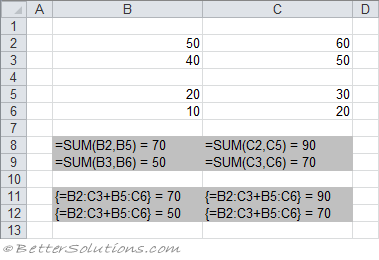# Sum cells using array formula

1) Adding up values [Using a multi cell array formula]
This example illustrates two methods that can be used to add two block of numbers.The formulas in rows 8 and 9 use the SUM worksheet function to add up the values.
The formulas in rows 11 & 12 use an array formula to add up the values and must be entered using (Ctrl + Shift + Enter). This array formula performs the necessary calculation and then returns a four-item array.
Excel inserts curly brackets {} around the formula to indicate that it is an array formula. These brackets cannot be entered manually.
The array formula "{=A1:B2+A4:B5}" returns an array containing four numbers.
The formula must therefore occupy four cells since you cannot display more than one number in a cell.